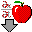# B5. Analysis of the General Michaelis-Menten Equation

The Michaelis-Menten equation can be simplified and studied under different conditions. First notice that $$(k_2 + k_3)/k_1$$ is a constant which is a function of relevant rate constants. This term is usually replaced by $$K_m$$ which is called the Michaelis constant (which was used in the Mathematica graph above). Likewise, when $$S$$ approaches infinity (i.e. $$S \gg K_m$$, equation 5 becomes $$v = k_3[E_o]$$ which is also a constant, called $$V_m$$ for maximal velocity. Substituting $$V_m$$ and $$K_m$$ into equation 5 gives the simplified equation:

$v = \dfrac{V_m[S]}{K_m+ [S]} \label{10}$

It is extremely important to note that $$K_m$$ in the general equation does not equal the $$K_s$$, the dissociation constant used in the rapid equilibrium assumption! $$K_m$$ and $$K_s$$ have the same units of molarity, however. A closer examination of $$K_m$$ shows that under the limiting case when $$k_2 \gg k_3$$ (the rapid equilibrium assumption) then,

$K_m = \dfrac{k_2 + k_3}{k_1} \approx \dfrac{k_2}{k_1} = K_d = K_s. \label{11}$

If we examine Equations \ref{9} and \ref{10} under several different scenarios, we can better understand the equation and the kinetic parameters:

• when $$S = 0$$, $$v = 0$$.
• when $$S \gg K_m$$, $$v = V_m = k_3E_o$$. (i.e. $$v$$ is zero order with respect to $$S$$ and first order in E. Remember, $$k_3$$ has units of s-1 since it is a first order rate constant. $$k_3$$ is often called the turnover number, because it describes how many molecules of $$S$$ "turn over" to product per second.
• $$v = \dfrac{V_m}{2}$$, when $$S = K_m$$.
• when $$S \ll K_m$$, $$v = \dfrac{V_mS}{K_m} = \dfrac{k_3E_oS}{K_m}$$ (i.e. the reaction is bimolecular, dependent on both on S and E. $$k_3/K_m$$ has units of M-1s-1, the same as a second order rate constant.

Notice that Equations \ref{9} and \ref{10} are exactly analysis to the previous equations we derived:

• $$ML = \dfrac{M_oL}{K_d + L}$$ for binding of $$L$$ to $$M$$
• $$J_o = \dfrac{J_m[A]}{K_d + [A]}$$ for rapid equilibrium binding and facilitated transport of $$A$$
• $$v_o = \dfrac{V_m[S]}{K_s + [S]}$$ for rapid equilibrium binding and catalytic conversion of $$A$$ to $$P$$.
• $$v_o = \dfrac{V_m[S]}{K_m+ [S]}$$ for steady state binding and catalytic conversion of $$A$$ to $$P$$.

Please notice that all these equations give hyperbolic dependencies of the y dependent variable ($$ML$$, $$J_o$$, and $$v_o$$) on the ligand, solute, or substrate concentration, respectively.Java Applet: Michaelis-Menten Plots